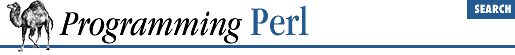home | O'Reilly's CD bookshelfs | FreeBSD | Linux | Cisco | Cisco Exam

#### 3.2.153 sort

```sort ```

SUBNAME
```
```

LIST
```

sort ```

BLOCK
```
```

LIST
```

sort ```

LIST
```
```

This function sorts the ``` LIST ``` and returns the sorted list value. By default, it sorts in standard string comparison order (undefined values sorting before defined null strings, which sort before everything else). ``` SUBNAME ``` , if given, is the name of a subroutine that returns an integer less than, equal to, or greater than 0, depending on how the elements of the list are to be ordered. (The handy ``` <=>``` and ``` cmp``` operators can be used to perform three-way numeric and string comparisons.) In the interests of efficiency, the normal calling code for subroutines is bypassed, with the following effects: the subroutine may not be a recursive subroutine, and the two elements to be compared are passed into the subroutine not via ``` @_``` but as \$a and \$b (see the examples below). The variables \$a and \$b are passed by reference, so don't modify them in the subroutine. ``` SUBNAME ``` may be a scalar variable name (unsubscripted), in which case the value provides the name of (or a reference to) the actual subroutine to use. In place of a ``` SUBNAME ``` , you can provide a ``` BLOCK ``` as an anonymous, in-line sort subroutine.

To do an ordinary numeric sort, say this:

```sub numerically { \$a <=> \$b; }
@sortedbynumber = sort numerically 53,29,11,32,7;```

To sort in descending order, simply reverse the ``` \$a``` and ``` \$b``` . To sort a list value by some associated value, use a hash lookup in the sort routine:

```sub byage {
\$age{\$a} <=> \$age{\$b};
}
@sortedclass = sort byage @class;```

As an extension of that notion, you can cascade several different comparisons using the handy comparison operators, which work nicely for this because when they return ``` 0``` they fall through to the next case. The routine below sorts to the front of the list those people who are first richer, then taller, then younger, then less alphabetically challenged. We also put a final comparison between ``` \$a``` and ``` \$b``` to make sure the ordering is always well defined.

```sub prospects {
\$money{\$b} <=> \$money{\$a}
or
\$height{\$b} <=> \$height{\$a}
or
\$age{\$a} <=> \$age{\$b}
or
\$lastname{\$a} cmp \$lastname{\$b}
or
\$a cmp \$b;
}
@sortedclass = sort prospects @class;```

To sort fields without regard to case, say:

`@sorted = sort { lc(\$a) cmp lc(\$b) } @unsorted;`

And finally, note the equivalence of the two ways to sort in reverse:

```sub backwards { \$b cmp \$a; }
@harry = qw(dog cat x Cain Abel);
@george = qw(gone chased yz Punished Axed);
print sort @harry;                   # prints AbelCaincatdogx
print sort backwards @harry;         # prints xdogcatCainAbel
print reverse sort @harry;           # prints xdogcatCainAbel
print sort @george, "to", @harry;    # Remember, it's one LIST.
# prints AbelAxedCainPunishedcatchaseddoggonetoxyz```

Do not declare \$a and \$b as lexical variables (with my ). They are package globals (though they're exempt from the usual restrictions on globals when you're using ``` use strict``` ). You do need to make sure your sort routine is in the same package though, or qualify ``` \$a``` and ``` \$b``` with the package name of the caller.

One last caveat. Perl's sort is implemented in terms of C's qsort (3) function. Some qsort (3) versions will dump core if your sort subroutine provides inconsistent ordering of values.